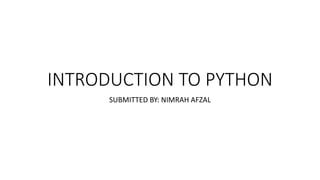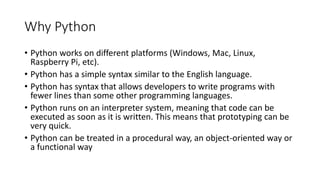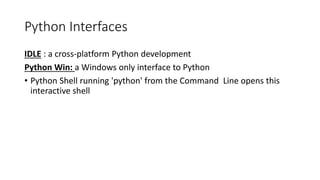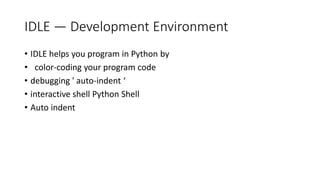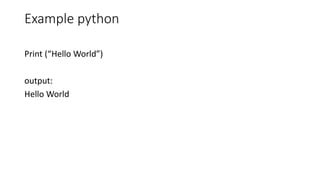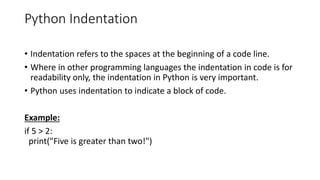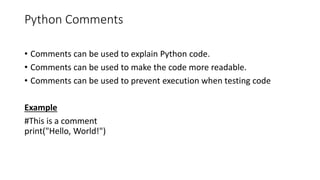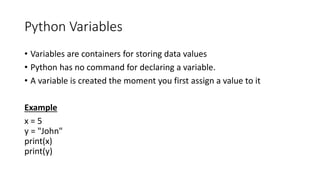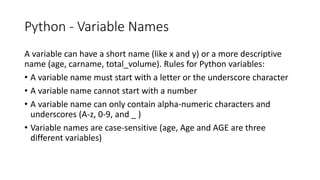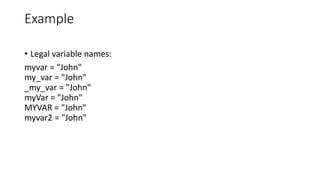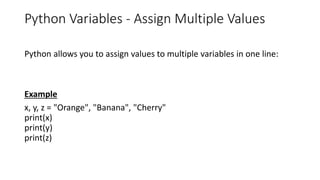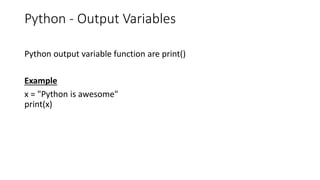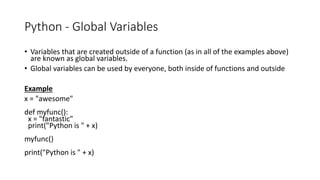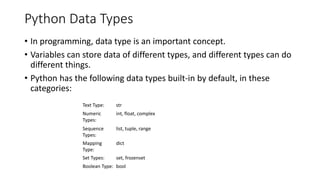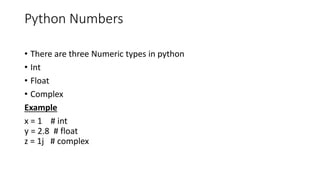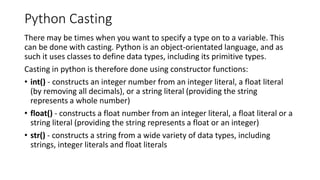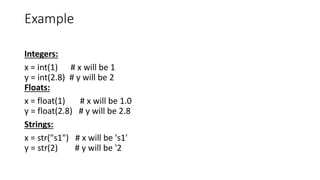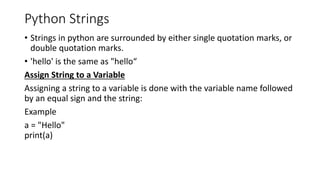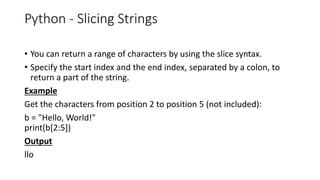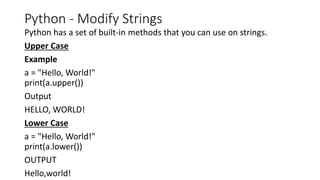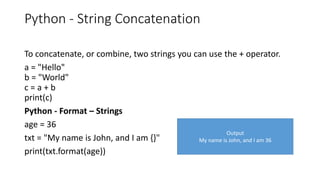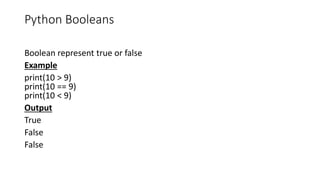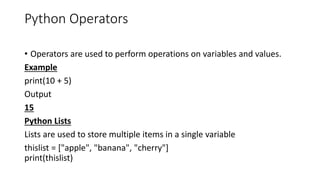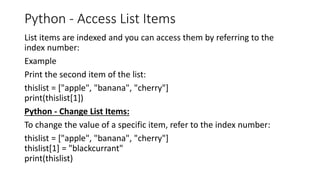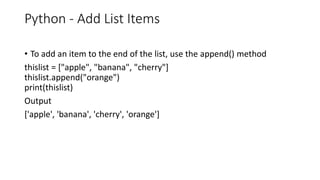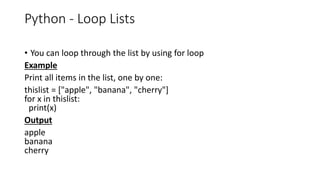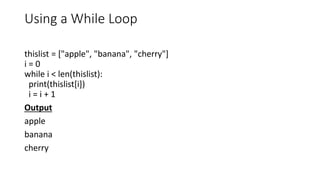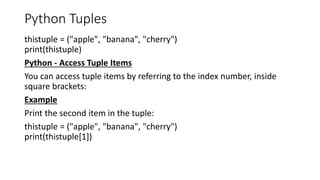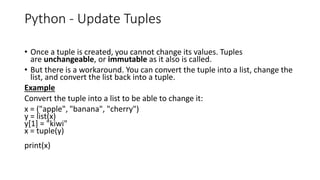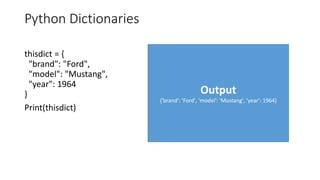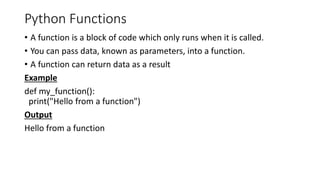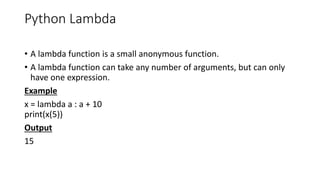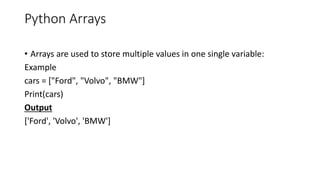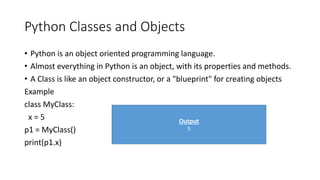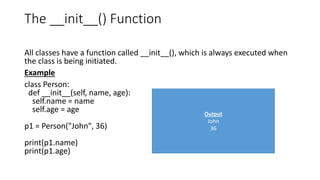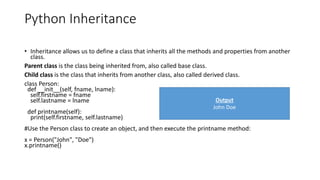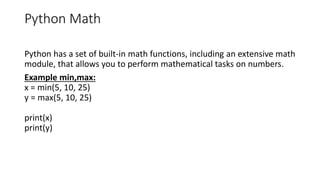1 de 38

### INTRODUCTION TO PYTHON.pptx

1. INTRODUCTION TO PYTHON SUBMITTED BY: NIMRAH AFZAL
2. Introduction to Python • Python is an interpreted, object-oriented, high-level programming language • Python's simple, easy to learn syntax emphasizes readability and therefore reduces the cost of program maintenance. • A standard distribution includes many modules • Dynamic typed Source can be compiled or run just-in-time Similar to perl, tcl, ruby
3. Why Python • Python works on different platforms (Windows, Mac, Linux, Raspberry Pi, etc). • Python has a simple syntax similar to the English language. • Python has syntax that allows developers to write programs with fewer lines than some other programming languages. • Python runs on an interpreter system, meaning that code can be executed as soon as it is written. This means that prototyping can be very quick. • Python can be treated in a procedural way, an object-oriented way or a functional way
4. Python Interfaces IDLE : a cross-platform Python development Python Win: a Windows only interface to Python • Python Shell running 'python' from the Command Line opens this interactive shell
5. IDLE — Development Environment • IDLE helps you program in Python by • color-coding your program code • debugging ' auto-indent ‘ • interactive shell Python Shell • Auto indent
6. Example python Print (“Hello World”) output: Hello World
7. Python Indentation • Indentation refers to the spaces at the beginning of a code line. • Where in other programming languages the indentation in code is for readability only, the indentation in Python is very important. • Python uses indentation to indicate a block of code. Example: if 5 > 2: print("Five is greater than two!")
8. Python Comments • Comments can be used to explain Python code. • Comments can be used to make the code more readable. • Comments can be used to prevent execution when testing code Example #This is a comment print("Hello, World!")
9. Python Variables • Variables are containers for storing data values • Python has no command for declaring a variable. • A variable is created the moment you first assign a value to it Example x = 5 y = "John" print(x) print(y)
10. Python - Variable Names A variable can have a short name (like x and y) or a more descriptive name (age, carname, total_volume). Rules for Python variables: • A variable name must start with a letter or the underscore character • A variable name cannot start with a number • A variable name can only contain alpha-numeric characters and underscores (A-z, 0-9, and _ ) • Variable names are case-sensitive (age, Age and AGE are three different variables)
11. Example • Legal variable names: myvar = "John" my_var = "John" _my_var = "John" myVar = "John" MYVAR = "John" myvar2 = "John"
12. Python Variables - Assign Multiple Values Python allows you to assign values to multiple variables in one line: Example x, y, z = "Orange", "Banana", "Cherry" print(x) print(y) print(z)
13. Python - Output Variables Python output variable function are print() Example x = "Python is awesome" print(x)
14. Python - Global Variables • Variables that are created outside of a function (as in all of the examples above) are known as global variables. • Global variables can be used by everyone, both inside of functions and outside Example x = "awesome" def myfunc(): x = "fantastic" print("Python is " + x) myfunc() print("Python is " + x)
15. Python Data Types • In programming, data type is an important concept. • Variables can store data of different types, and different types can do different things. • Python has the following data types built-in by default, in these categories: Text Type: str Numeric Types: int, float, complex Sequence Types: list, tuple, range Mapping Type: dict Set Types: set, frozenset Boolean Type: bool
16. Python Numbers • There are three Numeric types in python • Int • Float • Complex Example x = 1 # int y = 2.8 # float z = 1j # complex
17. Python Casting There may be times when you want to specify a type on to a variable. This can be done with casting. Python is an object-orientated language, and as such it uses classes to define data types, including its primitive types. Casting in python is therefore done using constructor functions: • int() - constructs an integer number from an integer literal, a float literal (by removing all decimals), or a string literal (providing the string represents a whole number) • float() - constructs a float number from an integer literal, a float literal or a string literal (providing the string represents a float or an integer) • str() - constructs a string from a wide variety of data types, including strings, integer literals and float literals
18. Example Integers: x = int(1) # x will be 1 y = int(2.8) # y will be 2 Floats: x = float(1) # x will be 1.0 y = float(2.8) # y will be 2.8 Strings: x = str("s1") # x will be 's1' y = str(2) # y will be '2
19. Python Strings • Strings in python are surrounded by either single quotation marks, or double quotation marks. • 'hello' is the same as "hello“ Assign String to a Variable Assigning a string to a variable is done with the variable name followed by an equal sign and the string: Example a = "Hello" print(a)
20. Python - Slicing Strings • You can return a range of characters by using the slice syntax. • Specify the start index and the end index, separated by a colon, to return a part of the string. Example Get the characters from position 2 to position 5 (not included): b = "Hello, World!" print(b[2:5]) Output llo
21. Python - Modify Strings Python has a set of built-in methods that you can use on strings. Upper Case Example a = "Hello, World!" print(a.upper()) Output HELLO, WORLD! Lower Case a = "Hello, World!" print(a.lower()) OUTPUT Hello,world!
22. Python - String Concatenation To concatenate, or combine, two strings you can use the + operator. a = "Hello" b = "World" c = a + b print(c) Python - Format – Strings age = 36 txt = "My name is John, and I am {}" print(txt.format(age)) Output My name is John, and I am 36
23. Python Booleans Boolean represent true or false Example print(10 > 9) print(10 == 9) print(10 < 9) Output True False False
24. Python Operators • Operators are used to perform operations on variables and values. Example print(10 + 5) Output 15 Python Lists Lists are used to store multiple items in a single variable thislist = ["apple", "banana", "cherry"] print(thislist)
25. Python - Access List Items List items are indexed and you can access them by referring to the index number: Example Print the second item of the list: thislist = ["apple", "banana", "cherry"] print(thislist) Python - Change List Items: To change the value of a specific item, refer to the index number: thislist = ["apple", "banana", "cherry"] thislist = "blackcurrant" print(thislist)
26. Python - Add List Items • To add an item to the end of the list, use the append() method thislist = ["apple", "banana", "cherry"] thislist.append("orange") print(thislist) Output ['apple', 'banana', 'cherry', 'orange']
27. Python - Loop Lists • You can loop through the list by using for loop Example Print all items in the list, one by one: thislist = ["apple", "banana", "cherry"] for x in thislist: print(x) Output apple banana cherry
28. Using a While Loop thislist = ["apple", "banana", "cherry"] i = 0 while i < len(thislist): print(thislist[i]) i = i + 1 Output apple banana cherry
29. Python Tuples thistuple = ("apple", "banana", "cherry") print(thistuple) Python - Access Tuple Items You can access tuple items by referring to the index number, inside square brackets: Example Print the second item in the tuple: thistuple = ("apple", "banana", "cherry") print(thistuple)
30. Python - Update Tuples • Once a tuple is created, you cannot change its values. Tuples are unchangeable, or immutable as it also is called. • But there is a workaround. You can convert the tuple into a list, change the list, and convert the list back into a tuple. Example Convert the tuple into a list to be able to change it: x = ("apple", "banana", "cherry") y = list(x) y = "kiwi" x = tuple(y) print(x)
31. Python Dictionaries thisdict = { "brand": "Ford", "model": "Mustang", "year": 1964 } Print(thisdict) Output {'brand': 'Ford', 'model': 'Mustang', 'year': 1964}
32. Python Functions • A function is a block of code which only runs when it is called. • You can pass data, known as parameters, into a function. • A function can return data as a result Example def my_function(): print("Hello from a function") Output Hello from a function
33. Python Lambda • A lambda function is a small anonymous function. • A lambda function can take any number of arguments, but can only have one expression. Example x = lambda a : a + 10 print(x(5)) Output 15
34. Python Arrays • Arrays are used to store multiple values in one single variable: Example cars = ["Ford", "Volvo", "BMW"] Print(cars) Output ['Ford', 'Volvo', 'BMW']
35. Python Classes and Objects • Python is an object oriented programming language. • Almost everything in Python is an object, with its properties and methods. • A Class is like an object constructor, or a "blueprint" for creating objects Example class MyClass: x = 5 p1 = MyClass() print(p1.x) Output 5
36. The __init__() Function All classes have a function called __init__(), which is always executed when the class is being initiated. Example class Person: def __init__(self, name, age): self.name = name self.age = age p1 = Person("John", 36) print(p1.name) print(p1.age) Output John 36
37. Python Inheritance • Inheritance allows us to define a class that inherits all the methods and properties from another class. Parent class is the class being inherited from, also called base class. Child class is the class that inherits from another class, also called derived class. class Person: def __init__(self, fname, lname): self.firstname = fname self.lastname = lname def printname(self): print(self.firstname, self.lastname) #Use the Person class to create an object, and then execute the printname method: x = Person("John", "Doe") x.printname() Output John Doe
38. Python Math Python has a set of built-in math functions, including an extensive math module, that allows you to perform mathematical tasks on numbers. Example min,max: x = min(5, 10, 25) y = max(5, 10, 25) print(x) print(y)